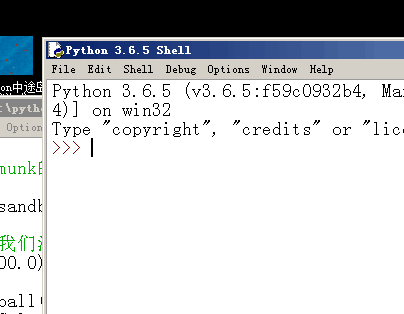# Python物理沙盒测试程序pyphysicssandbox```"""

"""
from``````import *

window("Python物理沙盒测试程序", 400, 300)
gravity(0.0, 500.0)                     #  设定重力参数

b1          = ball((100, 10), 30)
b1.color    = Color('green')
b1.friction = 0.25

b2       = static_ball((98, 100), 30)  # 静止的小球，接受碰撞检测
b2.color = Color('blue')

b3       = cosmetic_ball((198, 100), 50) # 装点门面的的小球,不接受碰撞检测
b3.color = Color('cyan')

box1       = static_rounded_box((0, 290), 400, 10, 3) # 最下面的地板，静止的
box1.color = Color('brown')

box2 = static_rounded_box((100,10), 50, 50, 5 ) # 上面品红色的圆角正方形
box2.color = Color('magenta')

tri1       = triangle((260, 35), (250, 35), (240, -15)) # 三角形
tri1.color = Color('red')

poly1       = polygon(((195, 35), (245, 35), (220, -15))) # 多边形
poly1.color = Color('blue')
poly1.wrap  = True

run()

print('完也！ ')
```

scratch